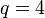# PGammaL(2,4) is isomorphic to S5

This article gives a proof/explanation of the equivalence of multiple definitions for the term symmetric group:S4
View a complete list of pages giving proofs of equivalence of definitions

## Statement

The projective semilinear group of degree two over field:F4 (the field of four elements) is isomorphic to symmetric group:S5.

In symbols:$P\Gamma L(2,4) \cong S_5$.

## Proof

Step no. Assertion/construction Facts used Previous steps used Explanation
1 For any field$k$, there is a natural faithful group action of$P\Gamma L(2,k)$ on$\mathbb{P}^1(k)$, the set of lines through the origin in$k^2$, and hence an injective group homomorphism from$P\Gamma L(2,k)$ to the symmetric group on$\mathbb{P}^1(k)$. Follows from definitions.
2 For$k$ the field of size$q$,$\mathbb{P}^1(k)$ has size$q + 1$ and thus the symmetric group on it has size$(q + 1)!$. Fact (1)
3 For$k$ the field of size$q = p^r$ with$p$ prime,$P\Gamma L(2,k) = P\Gamma L(2,q)$ has size$r(q^3 - q)$. Fact (2)
4 For$k$ the field of size$q = 4$,$P\Gamma L(2,k) =P\Gamma L(2,4)$ has order$2(4^3 - 3) = 2(60) = 120$ and$\operatorname{Sym}(\mathbb{P}^1(k))$ is the symmetric group of degree$4 + 1 = 5$ and has order$5! = 120$. Steps (2), (3) Plug in and evaluate.
5 For$k$ the field of size$q = 4$, the homomorphism of Step (1) gives an isomorphism from$P\Gamma L(2,4)$ to$S_5$. Steps (1), (4) By Steps (1) and (4), we get an injective homomorphism from$P\Gamma L(2,4)$ to$S_5$. Again by Step (4), both groups are finite and have the same order, hence the injective homomorphism must be an isomorphism.